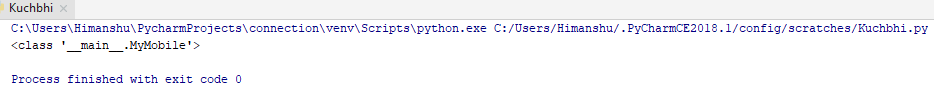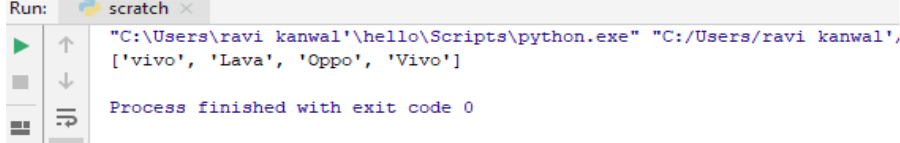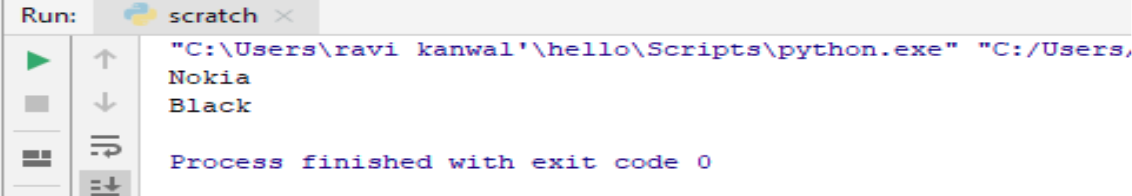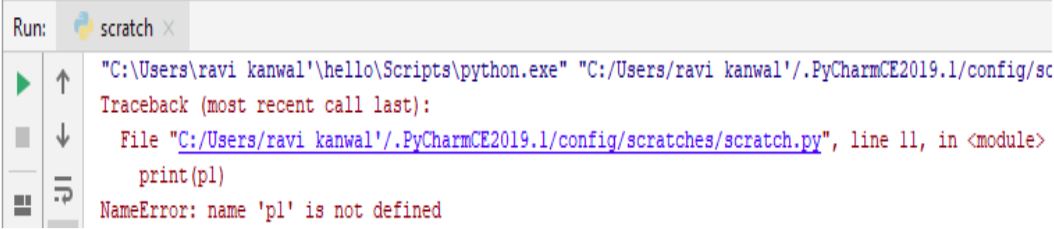×

Html & Html5

Introduction to Html

Component of Html

Html Form

Html5 basics

Html5 Grid

Html5 Graphics

Html5 Media

Html5 APIs

Python and Pandas

Introduction to Python

Component of Python

Python Classes/Objects

Python is an Object Oriented Programming Language (OOP). Object is collection of data . Python is an object and with it is properties and her method. A Class blueprint for the object.
let's have an example of creating a class:-

class MyMobile:

m = ["vivo","Lava","Oppo","Vivo"]

print(MyMobile)

Output of this ProgramOur class is been formed here .

Create an Object

We can use the class name to create a object. Any function object that is a class attributes defines the method for object of that class.
let's have an example of creating an object named m1 and print the value of minto it :-

class MyMobile:

m = ["vivo","Lava","Oppo","Vivo"]

m1=MyMobile()

print(m1.m)

Output of this ProgramOur object is been formed here .

The_init_() Function

The _init_() Function init is a constructor that mean create and sets up instance variables in the class.generally use for the initialize instance the given class.
let's have an example :-

class phone:

def __init__(mobile, name, color):

mobile.name = name

mobile.color = color

m1 = phone("Nokia", "Black")

print(m1.name)

print(m1.color)

Output of this ProgramHere this example we create the class of mobile and and the function_init_() to initialize the variables.and when we are create a new object than function _init_() automatically called.

Objects Methods

Object can also contains the method and the method in the object function that are belong to the object.The self() parameters is the reference the a current instance of the class and use access the variables in a class..
let's have an example of creating method in the Mobile class :-

class phone:

def __init__(self, name, age):

self.name = name

self.age = age

def myfunc(self):

print("Welcome to " + self.name)

m1 = phone("Nokia", "Black")

m1.myfunc()

Output of this ProgramIt does not have to be named self() whatever you just like to call and the first parameter of any function in the class.

Python Object Properties

We can change and modify the object properties in python .We also use and create the object and print the value of p1.color().
let's have an example :-

class phone:

def __init__(mob, name, color):

mob.name = name

mob.color = color

def mycell(mobile):

print("Hello this is my " + mobile.name)

p1 = phone("Nokia", "Black")

p1.color = "Black"

print(p1.color)

Output of this ProgramSo here through our object we just print the color of p1.

Delete Object

We use the del() keyword to delete the object in Python.
let's have an example :-

class phone:

def __init__(mob, name, color):

mob.name = name

mob.color = color

def mycell(mobile):

print("Hello this is my " + mobile.name)

p1 = phone("Nokia", "Black")

del p1

print(p1)

Output of this ProgramSo our object has been deleted that's why it gives us an error because 'p1' is not defined than.

python training insitute| Best IT Training classes in Gurgaon | python training